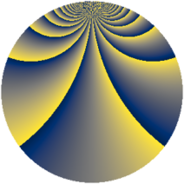# Properties

 Label 1183.2.bnLevel $1183$ Weight $2$ Character orbit 1183.bn Rep. character $\chi_{1183}(34,\cdot)$ Character field $\Q(\zeta_{52})$ Dimension $2832$ Sturm bound $242$

# Related objects

## Defining parameters

 Level: $$N$$ $$=$$ $$1183 = 7 \cdot 13^{2}$$ Weight: $$k$$ $$=$$ $$2$$ Character orbit: $$[\chi]$$ $$=$$ 1183.bn (of order $$52$$ and degree $$24$$) Character conductor: $$\operatorname{cond}(\chi)$$ $$=$$ $$1183$$ Character field: $$\Q(\zeta_{52})$$ Sturm bound: $$242$$

## Dimensions

The following table gives the dimensions of various subspaces of $$M_{2}(1183, [\chi])$$.

Total New Old
Modular forms 2928 2928 0
Cusp forms 2832 2832 0
Eisenstein series 96 96 0

## Trace form

 $$2832q - 48q^{2} - 52q^{4} - 18q^{7} - 56q^{8} + 176q^{9} + O(q^{10})$$ $$2832q - 48q^{2} - 52q^{4} - 18q^{7} - 56q^{8} + 176q^{9} - 44q^{11} - 38q^{14} - 48q^{15} + 156q^{16} - 44q^{18} - 26q^{21} - 96q^{22} - 52q^{25} - 6q^{28} - 52q^{29} - 156q^{30} - 296q^{32} - 10q^{35} - 52q^{36} - 64q^{37} - 196q^{39} - 34q^{42} - 52q^{43} - 168q^{44} + 28q^{46} - 26q^{49} - 24q^{50} - 52q^{51} - 12q^{53} - 26q^{56} - 44q^{57} - 8q^{58} - 96q^{60} - 176q^{63} - 52q^{64} - 12q^{65} + 304q^{67} + 178q^{70} - 52q^{71} - 24q^{72} + 204q^{74} - 26q^{77} - 96q^{78} - 68q^{79} - 216q^{81} - 22q^{84} - 272q^{85} - 400q^{86} - 260q^{88} + 6q^{91} + 156q^{92} - 24q^{93} - 52q^{95} + 2q^{98} - 64q^{99} + O(q^{100})$$

## Decomposition of $$S_{2}^{\mathrm{new}}(1183, [\chi])$$ into newform subspaces

The newforms in this space have not yet been added to the LMFDB.